## 跨周期均线压力支撑顶底趋势分析看操盘做单炒外汇现货黄金白银MTMT4平台

2019-11-05    来源：原创    编辑：翔博软件

0等于当前图表

M1=1

M5=5

M15=15

M30=30

H1=60

H4=240

D1=1440

W1=10080

MN=43200

MA1-MA5=均线周期

MA模式

Simple

Exponential

Smoothed

Linear wheghted

MA1颜色-MA5颜色=均线颜色显示

MA1字颜色-MA5字颜色=字体颜色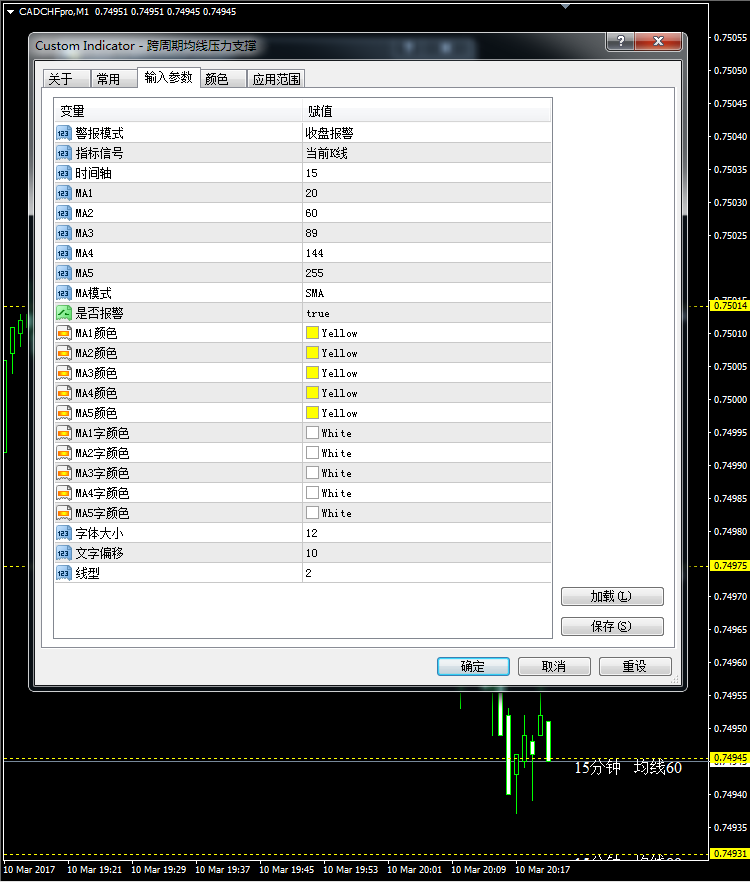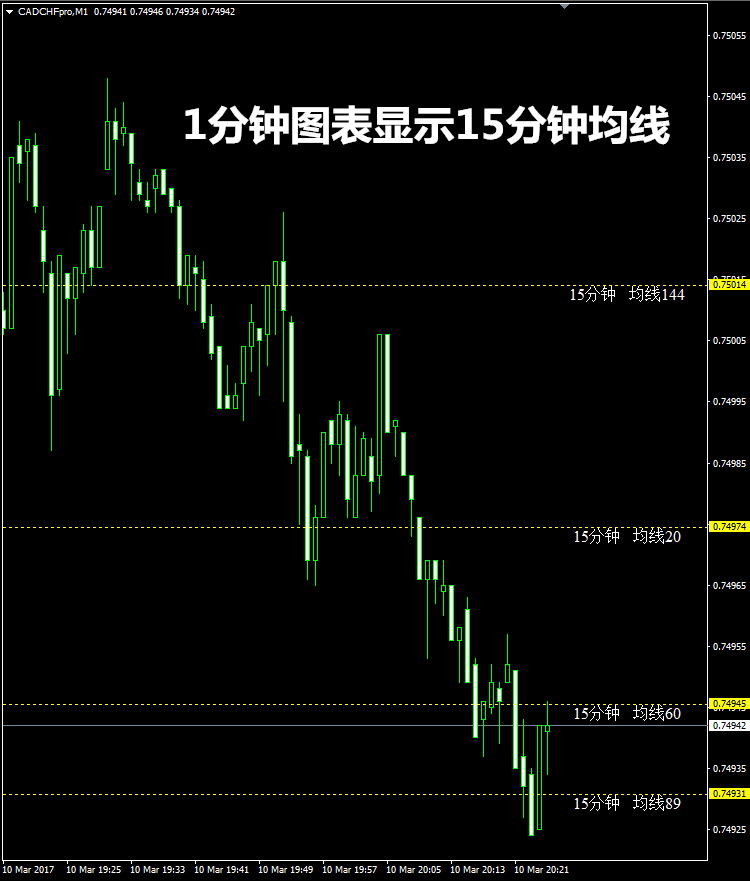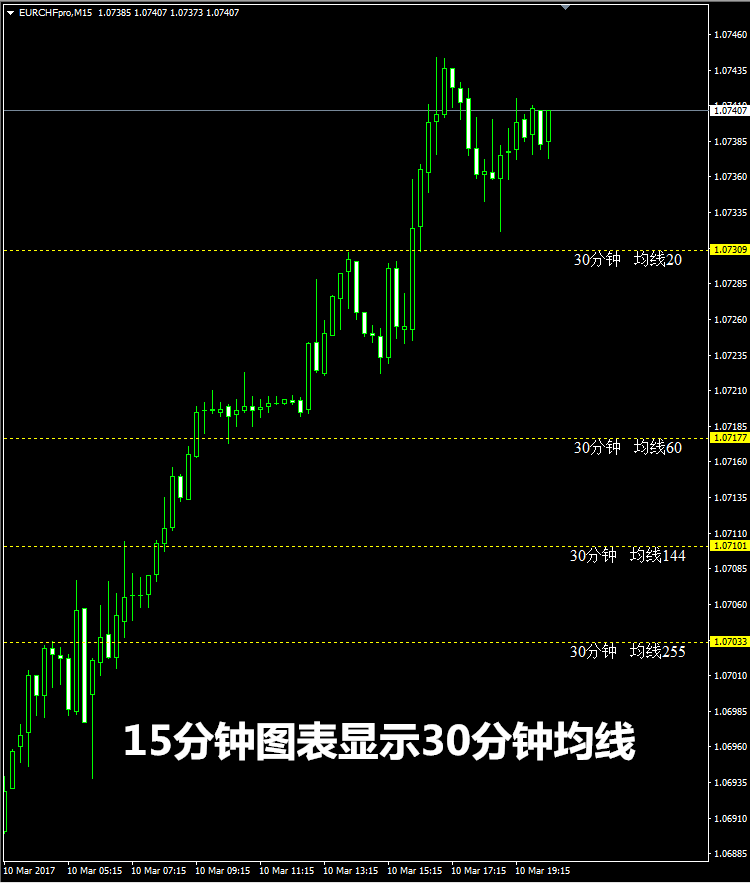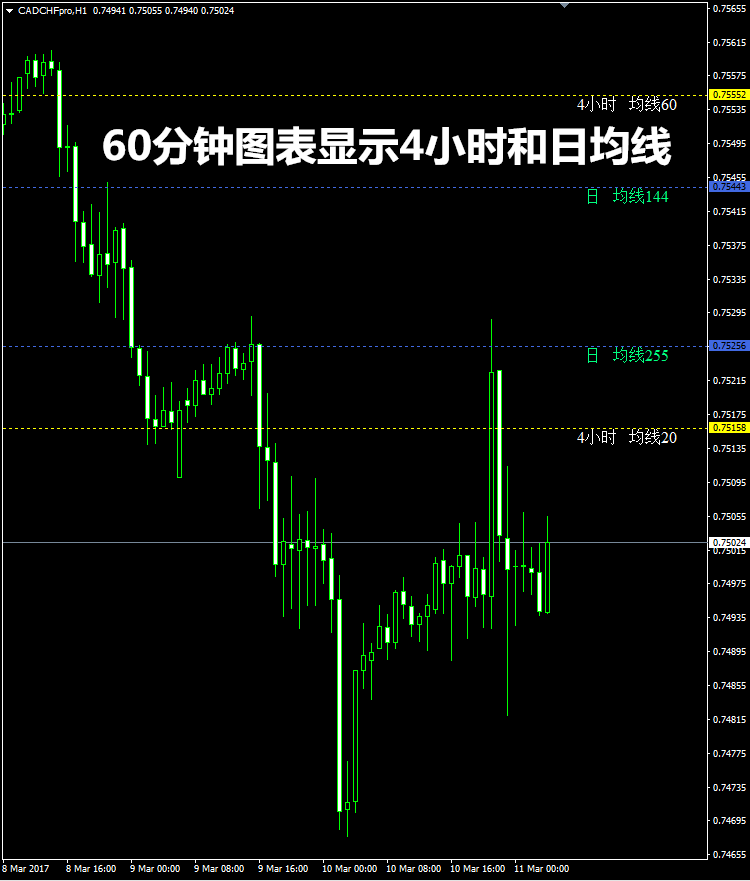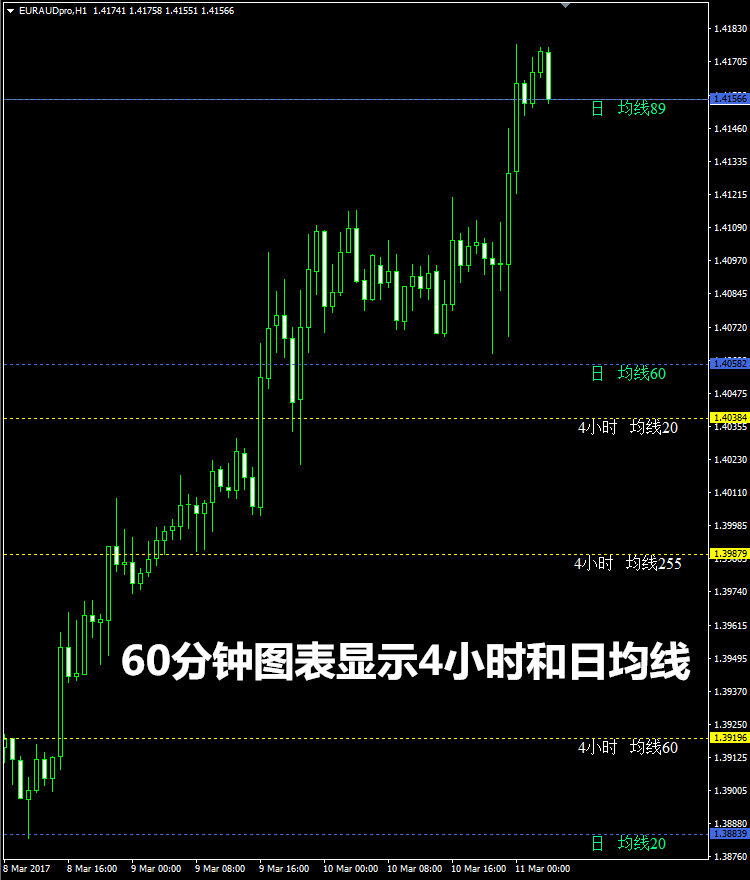1
3